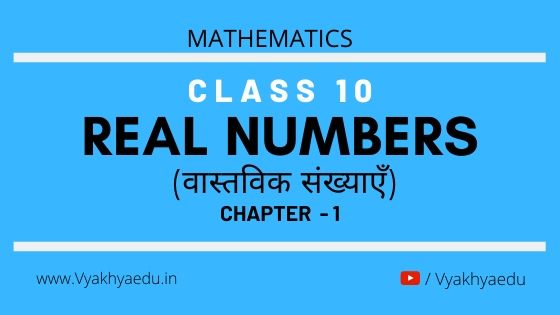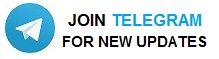### WATCH MORE VIDEOSReal Numbers (वास्तविक संख्याएँ)

 1.1 Introduction  1.2 Euclid’s Division Lemma  1.3 The Fundamental Theorem of Arithmetic  1.4 Revisiting Irrational Numbers 1.5 Revisiting Rational Numbers and Their Decimal Expansions  1.6 Summary

Introduction

Euclid’s division algorithm, as the name suggests, has to do with divisibility of integers. Stated simply, it says any positive integer a can be divided by another positive integer b in such a way that it leaves a remainder r that is smaller than b.

The Fundamental Theorem of Arithmetic, on the other hand, has to do something with multiplication of positive integers. You already know that every composite number can be expressed as a product of primes in a unique way—this important fact is the Fundamental Theorem of Arithmetic.

Euclid’s Division Lemma

Euclid’s division algorithm, as the name suggests, has to do with divisibility of integers. Stated simply, it says any positive integer a can be divided by another positive integer b in such a way that it leaves a remainder r that is smaller than b.

Theorem 1.1 (Euclid’s Division Lemma) : Given positive integers a and b, there exist unique integers q and r satisfying a = bq + r, 0 ≤ r < b.

where, integers q and r are called the quotient and remainder, respectively

Euclid’s division algorithm is a technique to compute the Highest Common Factor (HCF) of two given positive integers. Recall that the HCF of two positive integers a and b is the largest positive integer d that divides both a and b.

Example 1 : Use Euclid’s algorithm to find the HCF of 4052 and 12576.

Solution :

Step 1 : Since 12576 > 4052, we apply the division lemma to 12576 and 4052, to get

12576 = 4052 × 3 + 420

Step 2 : Since the remainder 420 ≠ 0, we apply the division lemma to 4052 and 420, to get

4052 = 420 × 9 + 272

Step 3 : We consider the new divisor 420 and the new remainder 272, and apply the division lemma to get

420 = 272 × 1 + 148

We consider the new divisor 272 and the new remainder 148, and apply the division lemma to get

272 = 148 × 1 + 124

We consider the new divisor 148 and the new remainder 124, and apply the division lemma to get

148 = 124 × 1 + 24

We consider the new divisor 124 and the new remainder 24, and apply the division lemma to get

124 = 24 × 5 + 4

We consider the new divisor 24 and the new remainder 4, and apply the division lemma to get

24 = 4 × 6 + 0

The remainder has now become zero, so our procedure stops. Since the divisor at this stage is 4, the HCF of 12576 and 4052 is 4.

Notice that 4 = HCF (24, 4) = HCF (124, 24) = HCF (148, 124) = HCF (272, 148) = HCF (420, 272) = HCF (4052, 420) = HCF (12576, 4052).

Euclid’s division algorithm is not only useful for calculating the HCF of very large numbers, but also because it is one of the earliest examples of an algorithm that a computer had been programmed to carry out.